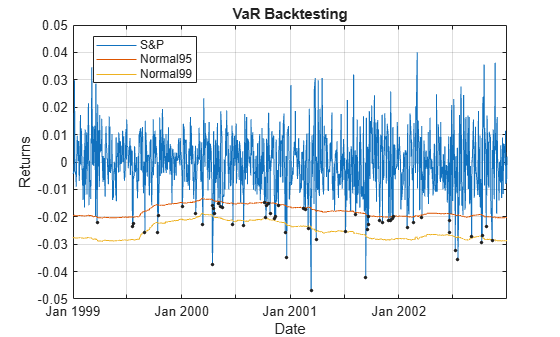# VaR Backtesting Workflow

This example shows a value-at-risk (VaR) backtesting workflow and the use of VaR backtesting tools. For a more comprehensive example of VaR backtesting, see Value-at-Risk Estimation and Backtesting.

### Step 1. Load the VaR backtesting data.

Use the `VaRBacktestData.mat` file to load the VaR data into the workspace. This example works with the `EquityIndex`, `Normal95`, and `Normal99` numeric arrays. These arrays are equity returns and the corresponding VaR data at 95% and 99% confidence levels is produced with a normal distribution (a variance-covariance approach). See Value-at-Risk Estimation and Backtesting for an example on how to generate this VaR data.

```load('VaRBacktestData') disp([EquityIndex(1:5) Normal95(1:5) Normal99(1:5)])```
``` -0.0043 0.0196 0.0277 -0.0036 0.0195 0.0276 -0.0000 0.0195 0.0275 0.0298 0.0194 0.0275 0.0023 0.0197 0.0278 ```

The first column shows three losses in the first three days, but none of these losses exceeds the corresponding VaR (columns 2 and 3). The VaR model fails whenever the loss (negative of returns) exceeds the VaR.

### Step 2. Generate a VaR backtesting plot.

Use the `plot` function to visualize the VaR backtesting data. This type of visualization is a common first step when performing a VaR backtesting analysis.

```plot(Date,[EquityIndex -Normal95 -Normal99]) title('VaR Backtesting') xlabel('Date') ylabel('Returns') legend('Returns','VaR 95%','VaR 99%')```### Step 3. Create a `varbacktest` object.

Create a `varbacktest` object for the equity returns and the VaRs at 95% and 99% confidence levels.

```vbt = varbacktest(EquityIndex,[Normal95 Normal99],... 'PortfolioID','S&P', ... 'VaRID',{'Normal95' 'Normal99'}, ... 'VaRLevel',[0.95 0.99]); disp(vbt)```
``` varbacktest with properties: PortfolioData: [1043x1 double] VaRData: [1043x2 double] PortfolioID: "S&P" VaRID: ["Normal95" "Normal99"] VaRLevel: [0.9500 0.9900] ```

### Step 4. Run a summary report.

Use the `summary` function to obtain a summary for the number of observations, the number of failures, and other simple metrics.

`summary(vbt)`
```ans=2×10 table PortfolioID VaRID VaRLevel ObservedLevel Observations Failures Expected Ratio FirstFailure Missing ___________ __________ ________ _____________ ____________ ________ ________ ______ ____________ _______ "S&P" "Normal95" 0.95 0.94535 1043 57 52.15 1.093 58 0 "S&P" "Normal99" 0.99 0.9837 1043 17 10.43 1.6299 173 0 ```

### Step 5. Run all tests.

Use the `runtests` function to display the final test results all at once.

`runtests(vbt)`
```ans=2×11 table PortfolioID VaRID VaRLevel TL Bin POF TUFF CC CCI TBF TBFI ___________ __________ ________ ______ ______ ______ ______ ______ ______ ______ ______ "S&P" "Normal95" 0.95 green accept accept accept accept accept reject reject "S&P" "Normal99" 0.99 yellow reject accept accept accept accept accept accept ```

### Step 6. Run individual tests.

After running all tests, you can investigate the details of particular tests. For example, use the `tl` function to run the traffic light test.

`tl(vbt)`
```ans=2×9 table PortfolioID VaRID VaRLevel TL Probability TypeI Increase Observations Failures ___________ __________ ________ ______ ___________ _______ ________ ____________ ________ "S&P" "Normal95" 0.95 green 0.77913 0.26396 0 1043 57 "S&P" "Normal99" 0.99 yellow 0.97991 0.03686 0.26582 1043 17 ```

### Step 7. Create VaR backtests for multiple portfolios.

You can create VaR backtests for different portfolios, or the same portfolio over different time windows. Run tests over two different subwindows of the original test window.

```Ind1 = year(Date)<=2000; Ind2 = year(Date)>2000; vbt1 = varbacktest(EquityIndex(Ind1),[Normal95(Ind1,:) Normal99(Ind1,:)],... 'PortfolioID','S&P, 1999-2000',... 'VaRID',{'Normal95' 'Normal99'},... 'VaRLevel',[0.95 0.99]); vbt2 = varbacktest(EquityIndex(Ind2),[Normal95(Ind2,:) Normal99(Ind2,:)],... 'PortfolioID','S&P, 2001-2002',... 'VaRID',{'Normal95' 'Normal99'},... 'VaRLevel',[0.95 0.99]);```

### Step 8. Display a summary report for both portfolios.

Use the `summary` function to display a summary for both portfolios.

```Summary = [summary(vbt1); summary(vbt2)]; disp(Summary)```
``` PortfolioID VaRID VaRLevel ObservedLevel Observations Failures Expected Ratio FirstFailure Missing ________________ __________ ________ _____________ ____________ ________ ________ ______ ____________ _______ "S&P, 1999-2000" "Normal95" 0.95 0.94626 521 28 26.05 1.0749 58 0 "S&P, 1999-2000" "Normal99" 0.99 0.98464 521 8 5.21 1.5355 173 0 "S&P, 2001-2002" "Normal95" 0.95 0.94444 522 29 26.1 1.1111 35 0 "S&P, 2001-2002" "Normal99" 0.99 0.98276 522 9 5.22 1.7241 45 0 ```

### Step 9. Run all tests for both portfolios.

Use the `runtests` function to display the final test result for both portfolios.

```Results = [runtests(vbt1);runtests(vbt2)]; disp(Results)```
``` PortfolioID VaRID VaRLevel TL Bin POF TUFF CC CCI TBF TBFI ________________ __________ ________ ______ ______ ______ ______ ______ ______ ______ ______ "S&P, 1999-2000" "Normal95" 0.95 green accept accept accept accept accept reject reject "S&P, 1999-2000" "Normal99" 0.99 green accept accept accept accept accept accept accept "S&P, 2001-2002" "Normal95" 0.95 green accept accept accept accept accept accept accept "S&P, 2001-2002" "Normal99" 0.99 yellow accept accept accept accept accept accept accept ```